# KSEEB SSLC Class 10 Maths Solutions Chapter 13 Statistics Ex 13.1

In this chapter, we provide KSEEB SSLC Class 10 Maths Solutions Solutions Chapter 13 Statistics Ex 13.1 for English medium students, Which will very helpful for every student in their exams. Students can download the latest KSEEB SSLC Class 10 Maths Solutions Solutions Chapter 13 Statistics Ex 13.1 pdf, free KSEEB SSLC Class 10 Maths Solutions Solutions Chapter 13 Statistics Ex 13.1 pdf download. Now you will get step by step solution to each question.

### Karnataka State Syllabus Class 10 Maths SolutionsChapter 13 Statistics Ex 13.1

Question 1.
A survey was conducted by a group of students as a part of their environment awareness programme, in which they collected the following data regarding the number of plants in 20 houses in a locality. Find the mean number of plants per house.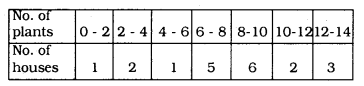Which method did you use for finding the mean, and why ?
Solution:
Average by Direct Method :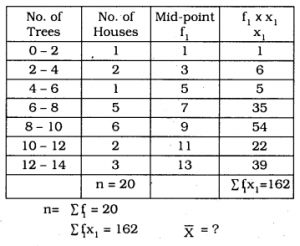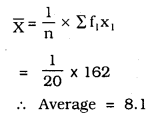Question 2.
Consider the following distribution of daily wages of 30 workers of a factory.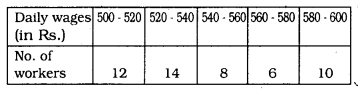Find the mean daily wages of the workers of the factory by using an appropriate method.
Solution:
Step deviation method :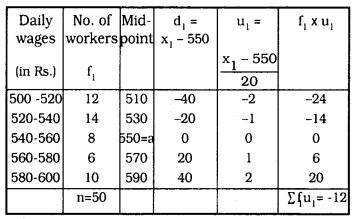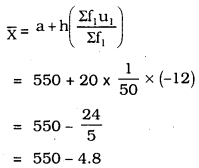∴ Average = 545.20.

Question 3.
The following distribution shows the daily pocket allowance of children of a locality. The mean pocket allowance is Rs. 18. Find the missing frequency f.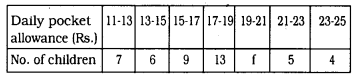Solution: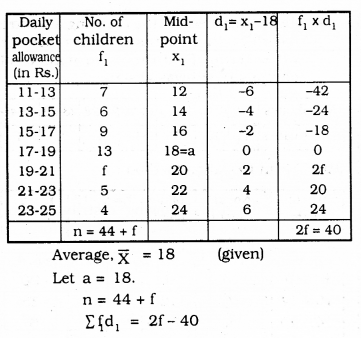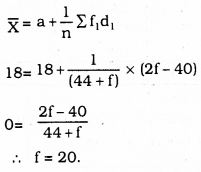Question 4.
Thirty women were examined in a hospital by a doctor and the number of heartbeats per minute were recorded and summarised as follows. Find the mean heartbeats per minute for these women, choosing a suitable method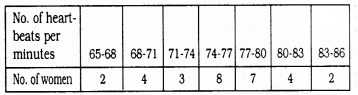Solution: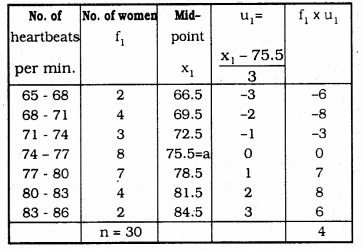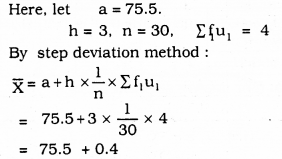∴ Mean heartbeats per minute = 75.9

Question 5.
In a retail market, fruit vendors were selling mangoes kept in packing boxes. These boxes contained varying number of mangoes. The following was the distribution of mangoes according to the number of boxes.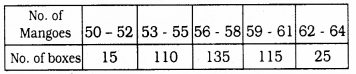Find the mean number of mangeos kept in a packing box. Which method of finding the mean did you choose ?
Solution:
Step Deviation Method :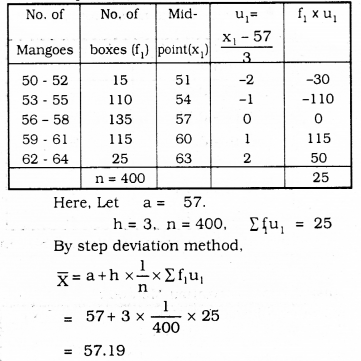∴ Mean Number of mangoes in the boxes = 57.19

Question 6.
The table below shows the daily expenditure on food of 25 households in a locality.
Find the mean daily expenditure on food by a suitable method.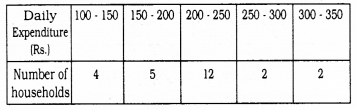Solution:
By Step deviation method: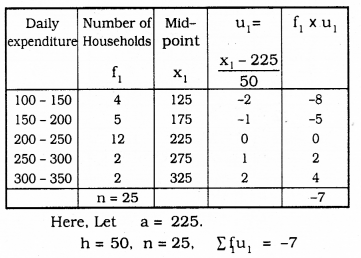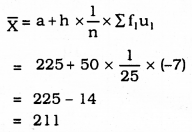∴ Mean Daily expenditure for food = Rs. 211.

Question 7.
To find out the concentration of SO2 in the air (in parts per million, i.e., ppm), the data was collected for 30 localities in a certain city and is presented below: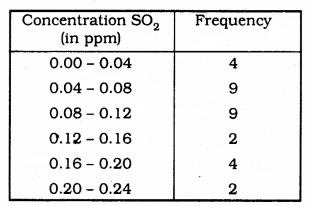Find the mean concentration of SO2 in the air.
Solution:
By step Deviation method: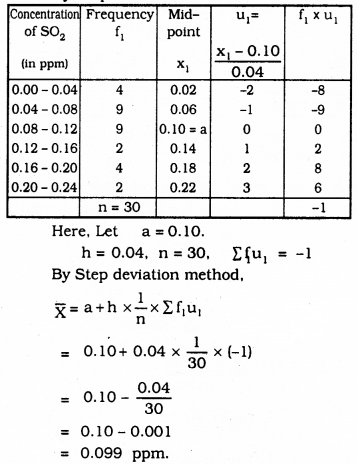∴ Mean Concentration of SO2 = 0.099 ppm.

Question 8.
A class teacher has the following absentee record of 40 students of a class for the whole term. Find the mean number of days a student was absent.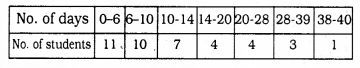Solution:
Mean Deviation method: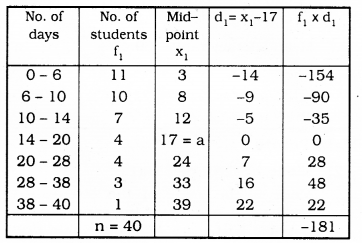Here, a = 17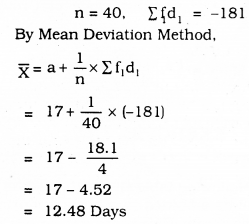∴ Mean number of days a student was absent = 12.48 Days.

Question 9.
The following table gives the literacy rate (in percentage) of 35 cities. Find the mean literacy rate.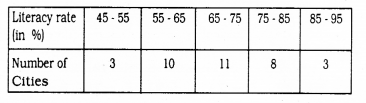Solution:
Step Deviation Method: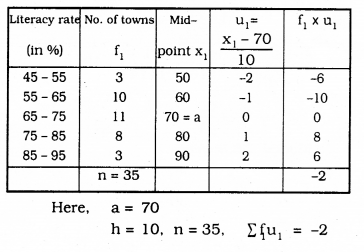By Step Deviation Method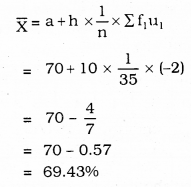∴ Mean Literacy rate = 69.43%

All Chapter KSEEB Solutions For Class 10 Maths

—————————————————————————–

All Subject KSEEB Solutions For Class 10

*************************************************

I think you got complete solutions for this chapter. If You have any queries regarding this chapter, please comment on the below section our subject teacher will answer you. We tried our best to give complete solutions so you got good marks in your exam.

If these solutions have helped you, you can also share kseebsolutionsfor.com to your friends.

Best of Luck!!